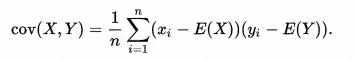# Covariance in fitting function

• I
• Silviu
In summary, the conversation discusses the calculation of covariance using a Python package called iminuit. The speaker is confused about the result they obtained for two different functions and is seeking clarification on the concept of covariance. They also mention the difference between covariance and correlation, stating that correlation is limited to 1 in magnitude.

#### Silviu

Hello! I have to calculate the covariance between 2 parameters from a fit function. I found this package in Python called iminuit that did a good fit and also calculate the covariance matrix of the parameters. I tested the package on a simple function and I am not sure I understand the result. When the function I put is x^2+y^2, which has the minimum for x=y=0, I obtain ((1.0, 0.0), (0.0, 1.0)), as a covariance matrix. When I use (x-y)^2 I obtain ((250.24975024975475, 249.75024975025426), (249.75024975025426, 250.24975024975475)), as a covariance matrix. I don't understand why do I get value of covariance greater than 1 and why in the first case I get 0 on the sides and 1 on the main diagonal? It is the first time I encounter covariance so I am not sure I got it right. Thank you!

The definition of covariance isIf two variable are independent we would expect in the limit of large n that the covariance would be 0. If they are dependent the covariance is not limited to 1.

Perhaps you are thinking of the correlation matrix call it Σ where the diagonal elements ∑II are always 1 and the off diagonal elements are -1 ≤ Σij ≤ 1

Your second function does not have an unique minimum but an infinitely long trough for values x=y.

covariance is not limited to 1. Correlation, the ratio of the covariance to the square root of the product of the variances, is limited (in magnitude) to 1.